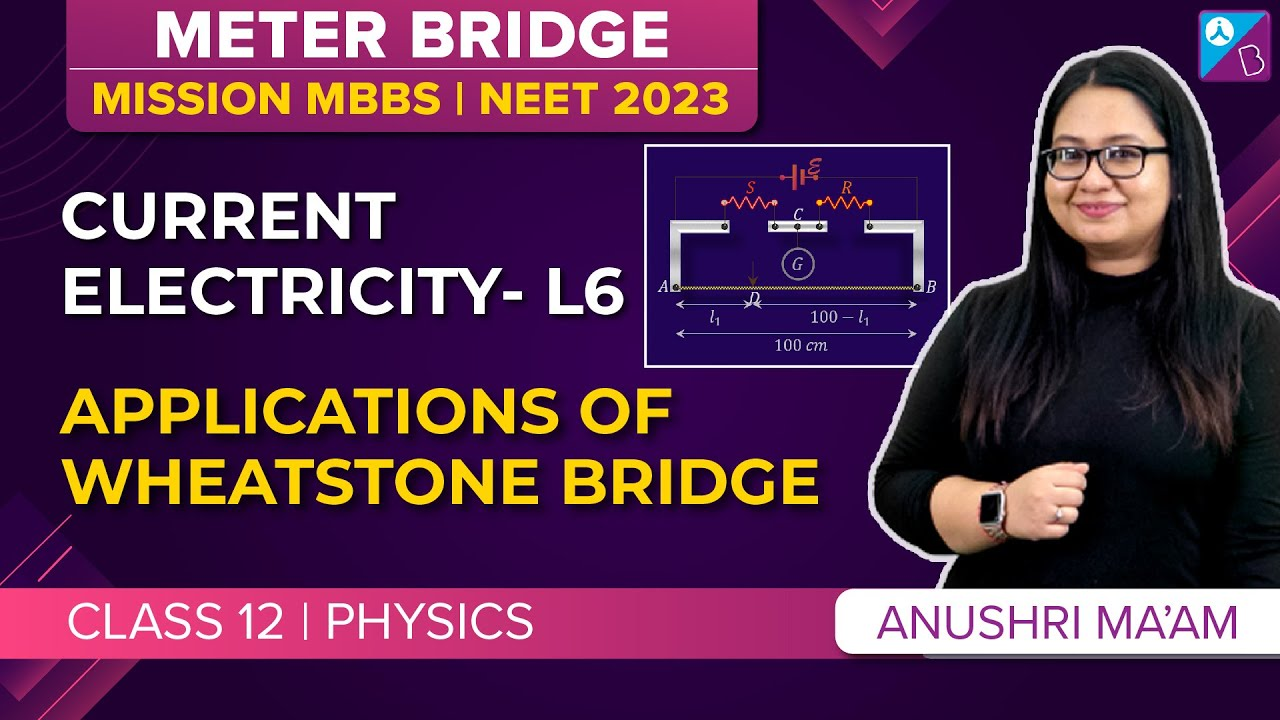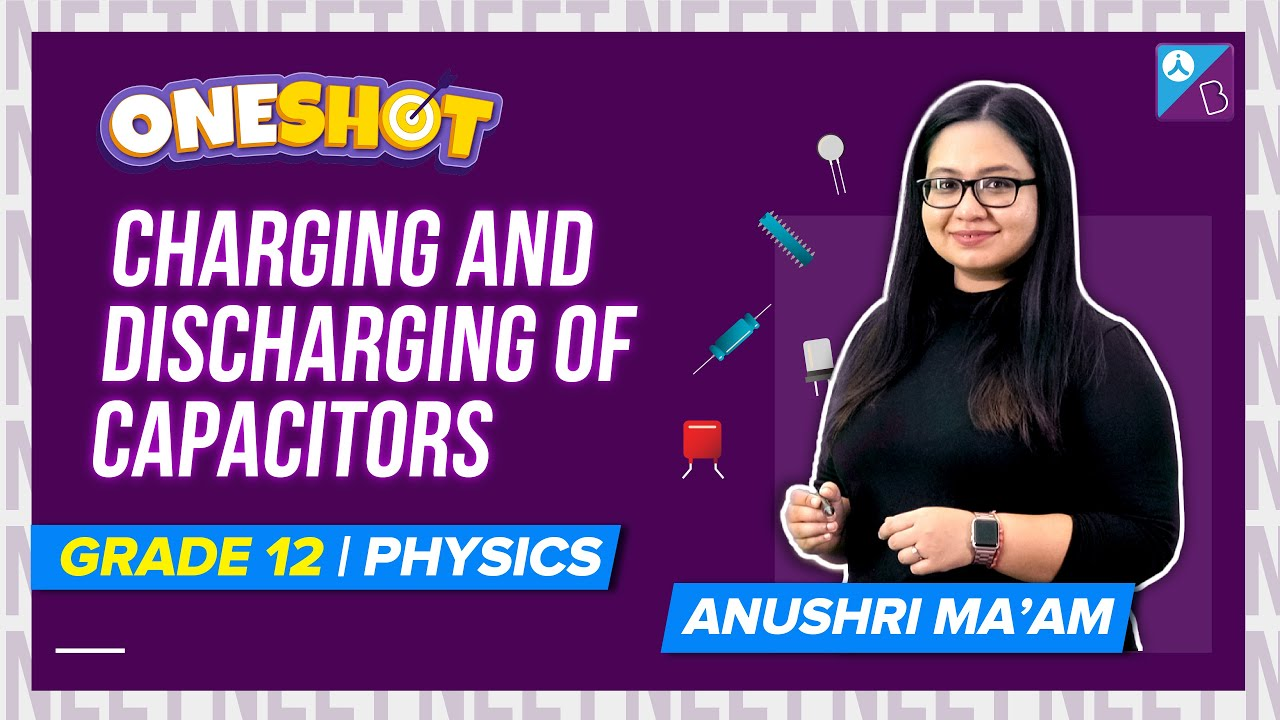Win up to 100% scholarship on Aakash BYJU'S JEE/NEET courses with ABNAT Win up to 100% scholarship on Aakash BYJU'S JEE/NEET courses with ABNAT

# Current Electricity MCQ for NEET

Current electricity or electric current is defined as the rate of flow of electric charges. In an electric circuit, these charges are carried by the electrons in the conductors. Ampere is the SI unit of electric current.

1. What does the internal resistance of a cell mean?

(a) The resistance of the material used in the cell

(b) The vessel of the cell

(c) The electrodes of the cell

(d) The electrolyte used in the cell

Answer: (d) The electrolyte used in the cell

2. A potentiometer is considered a versatile and accurate device to make an electrical measurement of EMF. Why?

(a) Because the method involves a combination

(b) There are cells involved

(d) As there is no flow of current through the galvanometer

Answer: (d) As there is no flow of current through the galvanometer

3. Consider copper and aluminium wire whose length and resistance are the same. Also, the specific resistance of aluminium is more than copper. Select the correct statement

(a) Copper and aluminium mass are the same

(b) Copper wire has more mass than the aluminium wire

(c) Copper wire has less mass than the aluminium wire

(d) The information given is incomplete

Answer: (d) The information given is incomplete

4. Following is the graph which represents the thermo EMF of two different metals which are joined end to end. One end of the metal is kept at a constant temperature while the other end is heated at very high temperature.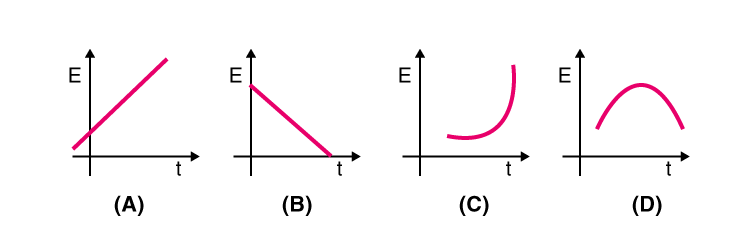5. Select the graph which depicts the variation in thermoelectric power and the temperature difference between the hot and cold junction in the thermocouples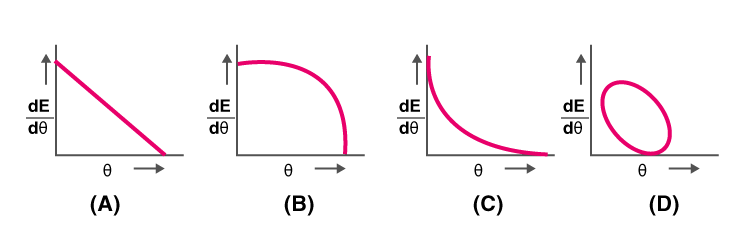6. Which of the following represents the relationship between the current flowing, i through the electric heater and the time taken, t.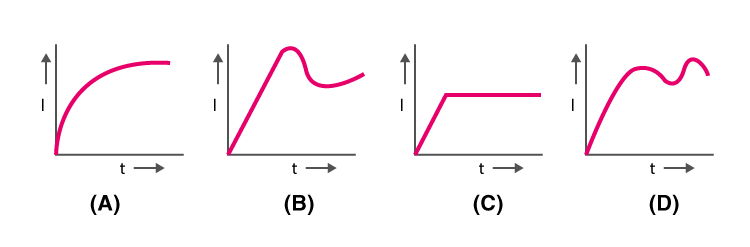7. Consider three voltmeters A, B, and C with resistance R, 1.5R, and 3R respectively. Potential difference is applied across the points X and Y, the voltmeter readings are VA, VB, and VC.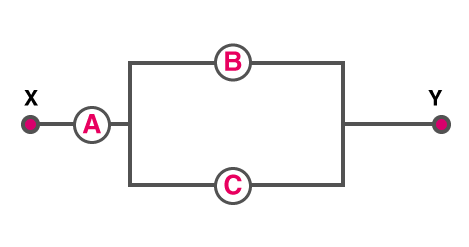(a) VA = VB ≠ VC

(b) VA ≠ VB ≠ VC

(c) VA = VB = VC

(d) VA ≠ VB = VC

Answer: (c) VA = VB = VC

8. What is the ratio of power dissipated if two electric bulbs are connected in series and the ratio of their resistance is 1:2?

(a) 1:4

(b) 1:2

(c) 2:1

(d) 1: 1

$$\begin{array}{l}9. Following \ is \ the \ Wheatstone \ bridge \ in \ which \ \frac{P}{Q}=\frac{R}{S}. \ Let \ K \ be \ the \ key \ which \ is \ closed, \ so \ what \ will \ be \ the \ deflection \ shown \ by \ galvanometer?\end{array}$$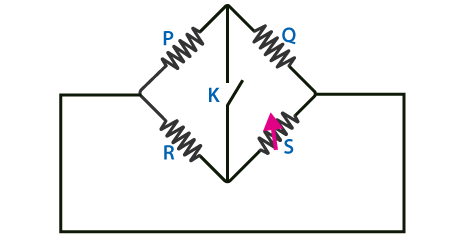(a) The deflection happens in either side

(b) There is no deflection

(c) Deflection happens on the right side

(d) Deflection happens on the left side

Answer: (a) The deflection happens in either side

10. What is the principle behind thermocouple

(a) Joule effect

(b) Thomson effect

(c) Seebeck effect

(d) Peltier effect

11. According to Faraday’s laws of electrolysis, to which of the following is the amount of decomposition proportional to?

(a) The electrochemical equivalent of the substance

$$\begin{array}{l}(b) \frac{1}{current}\end{array}$$
$$\begin{array}{l}(c) \frac{1}{electrochemical \ equivalent}\end{array}$$
$$\begin{array}{l}(d) \frac{1}{time \ for \ which \ current \ passes}\end{array}$$

Answer: (a) The electrochemical equivalent of the substance